2020 NBA Most Improved Player of the Year Odds
-5000
0.02 to 1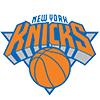Julius Randle
75.4% implied probability

+1300
13 to 1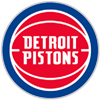Jerami Grant
5.5% implied probability

+2000
20 to 1Michael Porter
3.7% implied probability

+6000
60 to 1Nikola Jokic
1.3% implied probability

+6000
60 to 1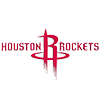Christian Wood
1.3% implied probability

+6000
60 to 1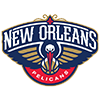Zion Williamson
1.3% implied probability

+8000
80 to 1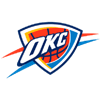Luguentz Dort
0.9% implied probability

+10000
100 to 1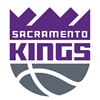De'Aaron Fox
0.8% implied probability

+10000
100 to 1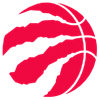Chris Boucher
0.8% implied probability

+10000
100 to 1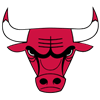Zach LaVine
0.8% implied probability

+15000
150 to 1Darius Bazley
0.5% implied probability

+15000
150 to 1Collin Sexton
0.5% implied probability

+15000
150 to 1Mikal Bridges
0.5% implied probability

+15000
150 to 1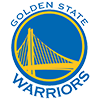Andrew Wiggins
0.5% implied probability

+15000
150 to 1Jarrett Allen
0.5% implied probability

+15000
150 to 1OG Anunoby
0.5% implied probability

+15000
150 to 1Jaylen Brown
0.5% implied probability

+15000
150 to 1Domantas Sabonis
0.5% implied probability

+15000
150 to 1RJ Barrett
0.5% implied probability

+15000
150 to 1Ja Morant
0.5% implied probability

+25000
250 to 1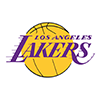Talen Horton-Tucker
0.3% implied probability

+25000
250 to 1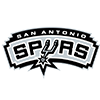Keldon Johnson
0.3% implied probability

+25000
250 to 1Deandre Ayton
0.3% implied probability

+25000
250 to 1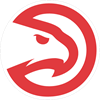Kevin Huerter
0.3% implied probability

+25000
250 to 1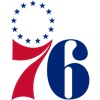Shake Milton
0.3% implied probability

+25000
250 to 1Lonzo Ball
0.3% implied probability

+25000
250 to 1Jayson Tatum
0.3% implied probability

+25000
250 to 1Dejounte Murray
0.3% implied probability

+25000
250 to 1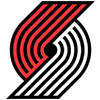Norman Powell
0.3% implied probability

+25000
250 to 1Devin Booker
0.3% implied probability

+25000
250 to 1Kevin Porter
0.3% implied probability

No changes have been recorded yet.